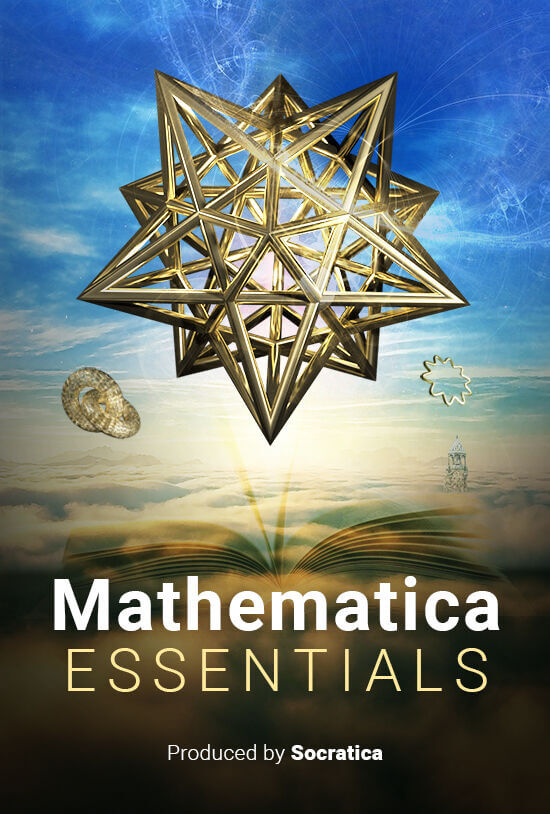# Mathematica Essentials

Mathematica Essentials is a comprehensive first course in Mathematica.  The name Mathematica may sounds like the program is just for mathematics, it can be used in ANY computational field: financial analysis, machine learning, data visualization, bioinformatics, speech recognition, and much, much more.

We will also cover the essential mathematical applications: solving equations, numerical methods, calculus, differential equations, linear algebra, modular arithmetic, and much more.  While doing so, we will cover the essential features of the Wolfram Language, the language that lies at the heart of Mathematica, Wolfram | One, and other products from Wolfram.# Exploring Houdini

Learn how to use Houdini, the powerful visual effects software by SideFX. We will start with the basics, and then expand into the more advanced features including Python, VEX, CHOPs, dynamics, RBD simulations, pyro sims, modelling techniques, and much more.